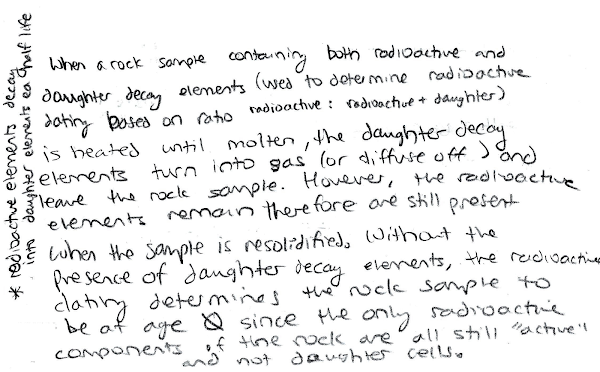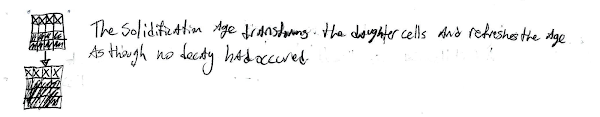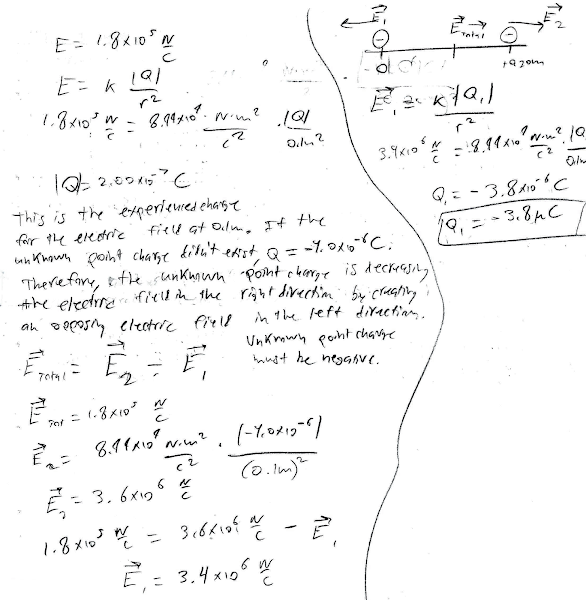## 20150624

### Physics final exam question: "resetting" solidification age of sample

Physics 205B Final Exam, spring semester 2015
Cuesta College, San Luis Obispo, CA

A rock sample with an extremely old solidification age (as determined by radioactive dating) is heated until it is molten, and then cooled back down to a solid. Discuss what happens to its solidification age (as determined by radioactive dating), and explain why this happens. Explain your reasoning using properties of radioactive decay.

• p:
Correct. Discusses:
1. the solidification age of a rock is determined by comparing the amount of gaseous decay products to the amount of unstable radioactive isotopes in the sample; a higher ratio of gaseous products to unstable isotopes corresponds to a sample that had solidified a long time ago; and
2. heating the rock until molten would release the gaseous decay products, such that when it cools back down to a solid, it would have nothing to compare to the unstable isotopes that remain in the sample, effectively giving it a zero solidification age.
• r:
As (p), but argument indirectly, weakly, or only by definition supports the statement to be proven, or has minor inconsistencies or loopholes. One of the two points (1)-(2) correct, other is problematic/incomplete.
• t:
Nearly correct, but argument has conceptual errors, or is incomplete. Only one of the two points (1)-(2) correct, other is missing, or both are problematic.
• v:
Limited relevant discussion of supporting evidence of at least some merit, but in an inconsistent or unclear manner. Some garbled attempt at applying properties of radioactive decay.
• x:
Implementation/application of ideas, but credit given for effort rather than merit. Approach other than that of applying properties of radioactive decay.
• y:
Irrelevant discussion/effectively blank.
• z:
Blank.
Sections 30882, 30883
Exam code: finalLd0c
p: 11 students
r: 13 students
t: 6 students
v: 10 students
x: 0 students
y: 1 student
z: 2 students

A sample "p" response (from student 3019):Another sample "p" response (from student 5080):A sample "v" response (from student 1107):### Physics final exam problem: interference of two in-phase Wi-Fi antennae

Physics 205B Final Exam, spring semester 2015
Cuesta College, San Luis Obispo, CA

Cf. Giambattista/Richardson/Richardson, Physics, 2/e, Problem 25.31"Wifi"
Jason Cole
youtu.be/6hcK9B4HHY8A commercially available wireless router[*] broadcasts at a 0.12 m wavelength from two vertical antennae spaced 0.18 m apart. Assume that the two antennae are in phase. Determine how many destructive interference (minima) directions there will be (if any) in the 360° range of all possible directions. Show your work and explain your reasoning using the properties of source phases, path lengths, and interference.

• p:
Correct. Approximates two in-phase antennae as a double slit, and equates path length difference approximation d⋅sinθ with destructive interference (minima) condition for in-phase sources to find θ = 19° and 90° as measured counterclockwise from the θ = 0° south direction, such that there are six unique directions of destructive interference in the 360° range of all possible directions. Okay if minima directions in the south-east quadrant are not correctly mapped via symmetry to find all minima directions, if at least the two unique θ = 19° and 90° directions in the southeast quadrant are found.
• r:
Nearly correct, but includes minor math errors. Only specifically searches over the cardinal directions, and finds of these that only east and west have destructive interference.
• t:
Nearly correct, but approach has conceptual errors, and/or major/compounded math errors.
• v:
Implementation of right ideas, but in an inconsistent, incomplete, or unorganized manner.
• x:
Implementation of ideas, but credit given for effort rather than merit. No clear attempt at applying path length differences and interference.
• y:
Irrelevant discussion/effectively blank.
• z:
Blank.
Sections 30882, 30883
Exam code: finalLd0c
p: 4 students
r: 13 students
t: 5 students
v: 11 students
x: 4 students
y: 3 students
z: 3 students

### Physics final exam problem: unknown charge contributing to total electric field

Physics 205B Final Exam, spring semester 2015
Cuesta College, San Luis Obispo, CA

Cf. Giambattista/Richardson/Richardson, Physics, 2/e, Problem 16.15Two point charges are held at fixed locations. An unknown charge is at the origin, and a –4.0 µC charge is at x = +0.20 m. The electric field at x = +0.10 m points to the right and has a magnitude of 1.8✕105 N/C. Determine the sign (±) and amount of the unknown charge (in either coulombs or µC) that is located at the origin. Show your work and explain your reasoning using properties of electric forces, fields, and vector superposition.

• p:
Correct. Determines that:
1. the electric field E1 at x = +0.10 m due to the Q1 = 4.0 μC charge at x = +0.20 m points to the right, but is more than the total electric field Etot at x = +0.10 m (that points to the right), thus the electric field E2 at x = +0.10 m due to the Q2 charge at the origin must point to the left, and so the charge Q2 must be negative as well;
2. from setting up vector superposition of the two oppositely directed electric fields E1 and E2 at x = +0.10 m, that the Q2 charge at the origin must be –3.8 μC.
• r:
Nearly correct, but includes minor math errors.
• t:
Nearly correct, but approach has conceptual errors, and/or major/compounded math errors. At least recognizes that there is superposition of two electric fields at x = +0.10 m that result in the given Etot there.
• v:
Implementation of right ideas, but in an inconsistent, incomplete, or unorganized manner. Misinterprets Etot at x = +0.10 m m to be the electric force exerted by Q1 on Q2, and then solves for Q1 from Coulomb's law.
• x:
Implementation of ideas, but credit given for effort rather than merit. No clear attempt at applying electric forces, fields, and vector superposition.
• y:
Irrelevant discussion/effectively blank.
• z:
Blank.
Sections 30882, 30883
Exam code: finalLd0c
p: 3 students
r: 2 students
t: 8 students
v: 19 students
x: 4 student
y: 3 students
z: 4 student

A sample "p" response (from student 8282):A sample "v" response (from student 9224):